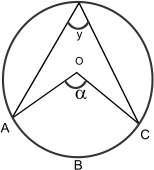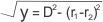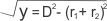# Quick Review: Circles

In this article, you will get an overview of the concepts and formulae related to Circles.
Consider the given circle:###### Important Terms
• Circumference: The distance around the circle is called circumference of the circle. It can be determined either by using the diameter d or the radius r.
C= 2πr or 2πd
• Arc: A part of a circle is known as an arc and is named according to its angle. Arcs are divided into 3 parts:
Minor Arcs: 00 < v < 1800
Major Arcs: 1800 < v < 3600
For Semi Circles: v = 1800
The length of the arc l is measured by the degree measure of the arc, v, and the circumference of the whole circle, C, by the following formula:
I = C*(V/360)
• Area: The area of the circle is calculated by the formula: πr2 As we know that radius, r =d/2. Therefore, we can also say that area, A = π(d2/4)
• Inscribed Angle: y = α/2
• Area of Sector: Area of Sector AOC y= πR2α/360
###### Important Points
• Triangle inscribed in a semi-circle = Right Triangle
• A circle is a polygon with infinite number of sides. If the perimeter is same, the polygon with the maximum number of sides has the maximum area. So, the circle has the maximum area for a given perimeter/circumference.
• If the radius of a circle is perpendicular to a chord, then it bisects the chord and vice versa.
• Equidistant chords from the center are equal in length.
• Tangents drawn to a circle from an exterior point are equal in length.
• If a square of maximum dimensions is cut from a circle, then the percentage area wasted is 36.3.
• If a circle of maximum radius is cut from a square, then the percentage area wasted is 21.5%.
• The length of the direct tangent to two circles of radii, r1 and r2 is ,where D is the distance between the two centers.
• The length of the transverse tangent to two circles of radii, r1 and r2 is• A circle is of 3600.